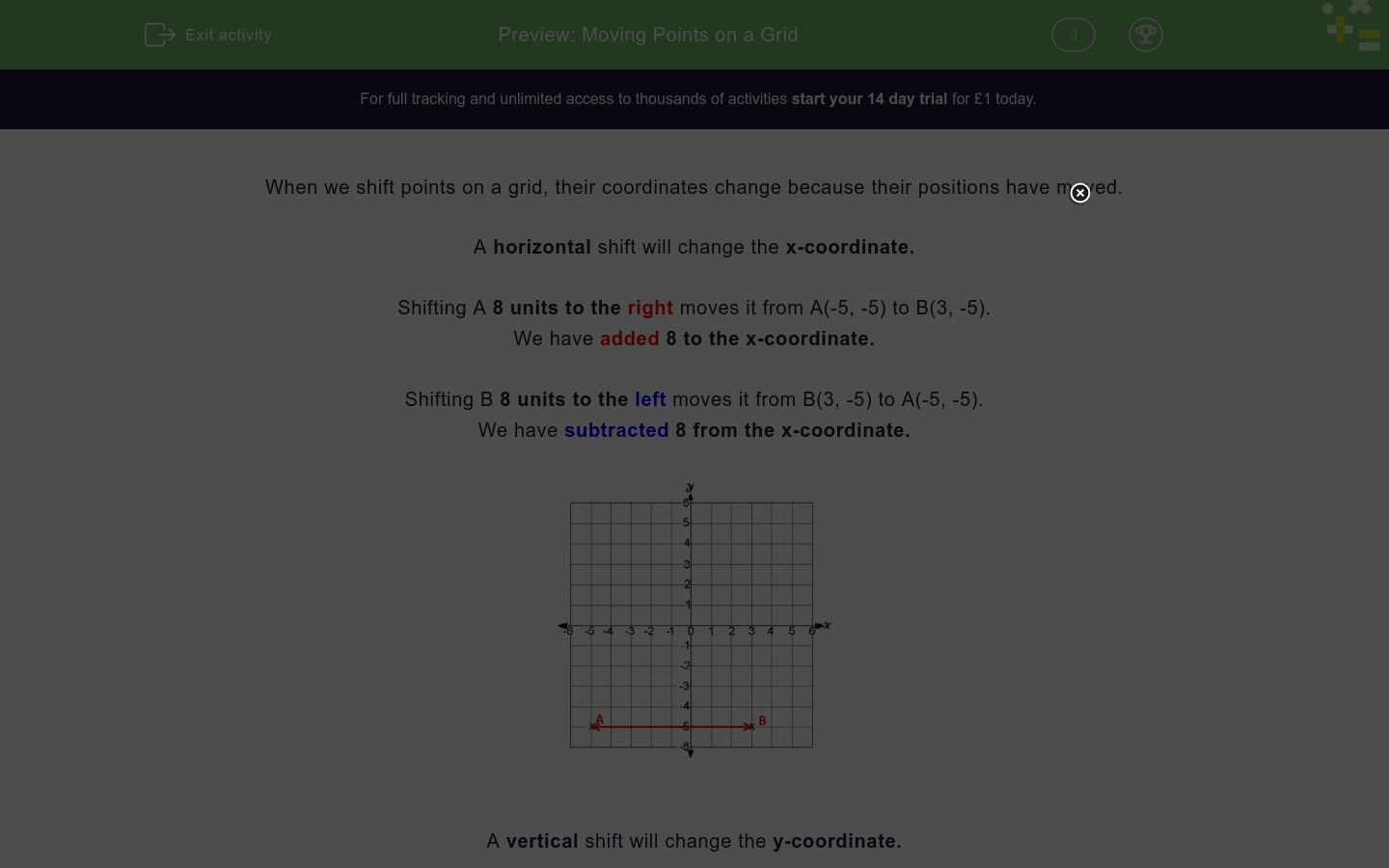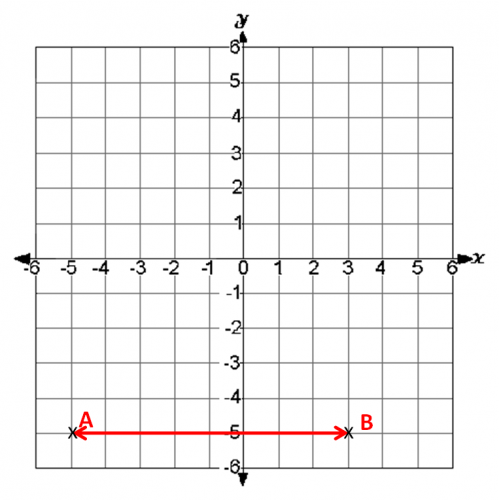# Moving Points on a Grid

In this worksheet, students must state the coordinates of points that have moved horizontally or vertically on a grid.Key stage:  KS 3

Curriculum topic:   Algebra

Curriculum subtopic:   Use Coordinates in All Four Quadrants

Difficulty level:### QUESTION 1 of 10

When we shift points on a grid, their coordinates change because their positions have moved.

A horizontal shift will change the x-coordinate.

Shifting A 8 units to the right moves it from A(-5, -5) to B(3, -5).

We have added 8 to the x-coordinate.

Shifting B 8 units to the left moves it from B(3, -5) to A(-5, -5).

We have subtracted 8 from the x-coordinate.A vertical shift will change the y-coordinate.

Shifting B 8 units up moves it from B(3, -5) to C(3, 3).

We have added 8 to the y-coordinate.

Shifting C 8 units down moves it from C(3, 3) to B(3, -5).

We have subtracted 8 from the y-coordinate.Look at this grid.

What are the coordinates of H after it has been shifted 5 units to the right?

(Write your answer in the form (a, b) using brackets and a comma between the coordinate numbers.)Look at this grid.

What are the coordinates of I after it has been shifted 5 units down?

(Write your answer in the form (a, b) using brackets and a comma between the coordinate numbers.)Look at this grid.

What are the coordinates of J after it has been shifted 9 units to the left?

(Write your answer in the form (a, b) using brackets and a comma between the coordinate numbers.)Look at this grid.

What are the coordinates of A after it has been shifted 5 units up?

(Write your answer in the form (a, b) using brackets and a comma between the coordinate numbers.)Look at this grid.

What are the coordinates of F after it has been shifted 7 units to the right?

(Write your answer in the form (a, b) using brackets and a comma between the coordinate numbers.)Look at this grid.

What are the coordinates of E after it has been shifted 4 units down?

(Write your answer in the form (a, b) using brackets and a comma between the coordinate numbers.)Look at this grid.

What are the coordinates of B after it has been shifted 3 units to the left?

(Write your answer in the form (a, b) using brackets and a comma between the coordinate numbers.)Look at this grid.

What are the coordinates of D after it has been shifted 7 units up?

(Write your answer in the form (a, b) using brackets and a comma between the coordinate numbers.)Look at this grid.

What are the coordinates of G after it has been shifted 6 units down?

(Write your answer in the form (a, b) using brackets and a comma between the coordinate numbers.)Look at this grid.

What are the coordinates of C after it has been shifted 7 units to the left?

(Write your answer in the form (a, b) using brackets and a comma between the coordinate numbers.)• Question 1

Look at this grid.

What are the coordinates of H after it has been shifted 5 units to the right?

(Write your answer in the form (a, b) using brackets and a comma between the coordinate numbers.)(2,5)
(2, 5)
EDDIE SAYS
5 is added to the x-coordinate.
-3 + 5 = 2.
• Question 2

Look at this grid.

What are the coordinates of I after it has been shifted 5 units down?

(Write your answer in the form (a, b) using brackets and a comma between the coordinate numbers.)(-1,0)
(-1, 0)
EDDIE SAYS
5 is taken away from the y-coordinate.
5 - 5 = 0.
• Question 3

Look at this grid.

What are the coordinates of J after it has been shifted 9 units to the left?

(Write your answer in the form (a, b) using brackets and a comma between the coordinate numbers.)(-6,5)
(-6, 5)
EDDIE SAYS
9 is taken away from the x-coordinate.
3 - 9 = -6.
• Question 4

Look at this grid.

What are the coordinates of A after it has been shifted 5 units up?

(Write your answer in the form (a, b) using brackets and a comma between the coordinate numbers.)(-5,2)
(-5, 2)
EDDIE SAYS
5 is added to the y-coordinate.
-3 + 5 = 2.
• Question 5

Look at this grid.

What are the coordinates of F after it has been shifted 7 units to the right?

(Write your answer in the form (a, b) using brackets and a comma between the coordinate numbers.)(4,3)
(4, 3)
EDDIE SAYS
7 is added to the x-coordinate.
-3 + 7 = 4.
• Question 6

Look at this grid.

What are the coordinates of E after it has been shifted 4 units down?

(Write your answer in the form (a, b) using brackets and a comma between the coordinate numbers.)(2,-5)
(2, -5)
EDDIE SAYS
4 is taken away from the y-coordinate.
-1 - 4 = -5.
• Question 7

Look at this grid.

What are the coordinates of B after it has been shifted 3 units to the left?

(Write your answer in the form (a, b) using brackets and a comma between the coordinate numbers.)(-4,-3)
(-4, -3)
EDDIE SAYS
3 is taken away from the x-coordinate.
-1 - 3 = -4.
• Question 8

Look at this grid.

What are the coordinates of D after it has been shifted 7 units up?

(Write your answer in the form (a, b) using brackets and a comma between the coordinate numbers.)(6,4)
(6, 4)
EDDIE SAYS
7 is added to the y-coordinate.
-3 + 7 = 4.
• Question 9

Look at this grid.

What are the coordinates of G after it has been shifted 6 units down?

(Write your answer in the form (a, b) using brackets and a comma between the coordinate numbers.)(2,-3)
(2, -3)
EDDIE SAYS
6 is taken away from the y-coordinate.
3 - 6 = -3.
• Question 10

Look at this grid.

What are the coordinates of C after it has been shifted 7 units to the left?

(Write your answer in the form (a, b) using brackets and a comma between the coordinate numbers.)(-4,-3)
(-4, -3)
EDDIE SAYS
7 is taken away from the x-coordinate.
3 - 7 = -4.
---- OR ----

Sign up for a £1 trial so you can track and measure your child's progress on this activity.

### What is EdPlace?

We're your National Curriculum aligned online education content provider helping each child succeed in English, maths and science from year 1 to GCSE. With an EdPlace account you’ll be able to track and measure progress, helping each child achieve their best. We build confidence and attainment by personalising each child’s learning at a level that suits them.

Get started